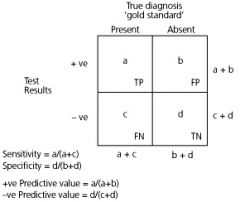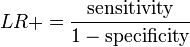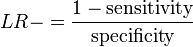• Shuffle
Toggle On
Toggle Off
• Alphabetize
Toggle On
Toggle Off
• Front First
Toggle On
Toggle Off
• Both Sides
Toggle On
Toggle Off
Toggle On
Toggle Off
Front

### How to study your flashcards.

Right/Left arrow keys: Navigate between flashcards.right arrow keyleft arrow key

Up/Down arrow keys: Flip the card between the front and back.down keyup key

H key: Show hint (3rd side).h key

A key: Read text to speech.a keyPlay buttonPlay buttonProgress

1/73

Click to flip

### 73 Cards in this Set

• Front
• Back
 What is prevalence? # of existing cases in the population at a specific time What is incidence? # of new cases in the disease-free population that developed over a period of time equation for prevalence total # of cases at 1 pt in time / TOTAL population equation for incidence # of new cases over a given period / total population AT RISK How are prevalence and incidence related? P = I x average duration of disease (D) When calculating incidence, how do you adjust the number for total population at risk? remember to subtract the # of new cases from the total population at risk bc these individuals are no longer at risk what kind of study can measure incidence? cohort study(longitudinal study --> follows a group of people who do not have the disease, but have risk factors) what kind of study can measure prevalence cross-sectional study(cross-section in time)AKA "prevalence study" what happens to the prevalence as the mortality of a disease decreases people live longer, so prevalence increases, bc the duration of the disease increased(think HIV pts getting treated and living longer)REMEMBER: P = I x D what is a cross-sectional study people in a population are examined for the presene of a disease of interest at a given point in time what are the advantages of cross-sectional studies 1provide an efficient means of examining a population, allowing cases and noncases to be assessed all at once2they can be used as a basis for diagnostic testing3they can be used to plan which health services to offer and where what are disadvantages of cross-sectional study 1one cannot determine causal relationships bc information is obtained only at a single point in time2the risk or incidence of disease cannot be directly measured SENSITIVITY VS SPECIFICITY"SNOUT" sensitive tests rule OUT disease SENSITIVITY VS SPECIFICITY"SPIN" specific tests rule IN disease How do you calculate:SensitivitySpecificityPPVNPVDefine sensitivity. probability that a patient with a disease will have a + test resulta sensitive test will rarely miss people what is the false negative ratio of a sensitivity test False Neg Ratio = 1 - Sensitivity Define specificity. probability that a patient without a disease will have a neg testa specific test will rarely determine that someone has the disease when in fact they do not what is the false positive ratio of a specificity test False Pos Ratio = 1 - Specificity What is the ideal diagnostic test in terms of sensitivity and specificity the ideal test will be high in both when is it desirable to have a high specificity diagnostic test when there's a big penalty for missing the diseaseorwhen it is desirable to EARLY IN THE DIAGNOSTIC WORKUPR OR SCREENING TEST to reduce a broad differential ie a diagnostic test for HIV/AIDS when is a high specificity test desirable to confirm a likely diagnosisorfor situations in which the false positive result may prove harmfulie a positive western blot confirmatory test for HIV what is the purpose of predictive values once a test result comes back, the results must be interpreted through use of predictive values (or posttest probabilities) Define PPV. the probability that a patient with a positive test result TRULY HAS the disease How is specificity and PPV related? the higher the specificity for a test, the higher it's PPV how is disease prevalence related to PPV the higher the disease prevalence, the higher the PPV of the test Define NPV. probability that a patient with a negative test result TRULY DOES NOT have teh disease How is sensitivity of a test related to NPV the more sensitive a test, the higher its NPV How is disease prevalence related to NPV? the lower the disease prevalence, the higher the NPV because prevalence and predictive value are related, it is advantageous to apply diagnostic tests to what patient population? diagnostic tests work better on individuals who have an increased likelihood of having the disease(ie someone who is at-risk) what happens to PPV and NPV when prevalence decreases PPV decreasesNPV increasesremember that if prevalence is low, even a test with high sensitivity or specificity will have a low PPV Besides predictive values, what's another way to measure the performance of a diagnostic test? likelihood ratio (LR) What is likelihood ratios (LR)? In evidence-based medicine, likelihood ratios are used for assessing the value of performing a diagnostic test. They use the sensitivity and specificity of the test to determine whether a test result usefully changes the probability that a condition (such as a disease state) exists. what is the likelihood ratio of a positive test resultthe probability of a person who has the disease testing positive divided by the probability of a person who does not have the disease but tests positive what is the likelihood ratio of a negative test resultthe probability of a person who has the disease but tests negative divided by the probability of a person who does not have the disease and tests negative How does pretest odds, post test odds and likelihood ratio relate to each other? The pretest odds of a particular diagnosis, multiplied by the likelihood ratio, determines the post-test odds. How do you interpret the likelihood ratio in medicine? A likelihood ratio of greater than 1 indicates the test result is associated with the disease.A likelihood ratio less than 1 indicates that the result is associated with absence of the disease. What does it mean for a likelihood ratio to be close to 1? Tests where the likelihood ratios lie close to 1 have little practical significance as the post-test probability (odds) is little different from the pre-test probability, and as such is used primarily for diagnostic purposes, and not screening purposes. How does the positive likelihood ratio change your odds of having a disease? the likelihood ratio of a positive test shows how much the odds of a disease are increased if the test result is positive How does the negative likelihood ratio change your odds of having a disease? the likelihood ratio of a negative tests shows how much the odds of a disease are decreased if the test result is negative What is pre-test odds? In summary, the pre-test probability refers to the chance that an individual has a disorder prior to the use of a diagnostic test. It allows the clinician to better interpret the results of the diagnostic test and helps to predict the likelihood of a true positive result. Describe how people are selected and followed in a cohort study. assemble a group of people who do not have the disease, but could potentially get itfor each risk factor for the disease, determine if the people in the group are exposed or unexposedthen follow the group over time and note the incidence of outcome events compared between the 2 groups 4 advantages of cohort studies 1the follow the same logic as the clinic question(if ppl are exposed, will they get the disease?)2they are the only way to DIRECTLY DETERMINE INCIDENCE (bc they follow the cohort over time to assess disease development)3they can be used to assess the relationship of a given exposure to many diseases4in prospective studies, exposure is elicited without bias from a knowing outcome 3 disadvantages of cohort studies 1they can be time consuming and expensive2studies assess only the relationship of the disease to a few exposure risk factors recorded at the start of the study3they require many subjects, which makes it difficult to study rare diseases Are cohort studies prospective or retrospective studies? they can be either one:they can be prospective in which a cohort is assembled in the present and followed into the futurethey can be retrospective, in which a cohort group is identified from past records and is followed to the present In a cohort study, what statistical test can you calculate? relative riskRRby following a cohort group over time and observing for disease development, the researcher can determine the Relative Risk ratio What does a case-control study analyze? the population How is a case-control study designed? a series of CASES are identified and a set of CONTROLS are sampled from the underlying population In a case-control study, what statistical test can you calculate/ you can estimate the frequency of exposure in the population at risk of the outcomethe researcher compares the frequency of exposure to a possible risk factor in the 2 groups How do you select the case and control to minimize differences? cases and controls should be comparable in terms of opportunity for exposurethey should be members from the same base population with an equal opportunity of risk factor exposure What is confounding? these are variables that influence the magnitude of a risk of getting a disease How do you control a confounding variable in a case-control? MATCHINGif somebody wanted to study the cause of myocardial infarct and thinks that the age is a probable confounding variable, each 67 years old infarct patient will be matched with a healthy 67 year old "control" person.EXCLUDE ITthe easiest way is to exclude it(ie if alcohol has a negative impact on heart disease, choose pts without alcoholism) advantages of case-control studies they use smaller groups than cohorts, thereby reducing costthey can be used to study rare diseases and can easily examine multiple risk factorx disadvantages of case-control studies 1studies cannot calculate disease prevalence or incidence or directly estimate relative risk bc the numbers of subjects with and without a disease are determined artificially by the investigator rather than by naturehowever, an odds ratio can be used to estimate a measure of relative risk (rate ratio)2retrospective data may be inaccurate owing to recall or survivorship biases Define absolute risk. its the incidence of disease What is attributable risk (or risk difference)? the difference in risk between the exposed and unexposed groups Equation for attributable risk. Attributable risk = incidence of disease in exposed - incidence in unexposed Define relative risk (or risk ratio). expresses how much more likely an exposed person is to get the disease in comparison to an unexposed person What does relative risk say about the relationship between exposure and disease? the relative risk indicates the relative strength of the association btw exposure and diseasethis is useful when considering what is causing the disease What is odds ratio? this is just an ESTIMATE of relative risk that is calculated in case-control studies (bc it can't be calculated directly) What does odds ratio say about one's exposure to a risk factor? the odds ratio tells how much more likely it is that a person with a disease has been exposed to a risk factor than someone without the disease What does odds ratio say about a person's chances of getting a disease in a case-control study? in case-control studies, the odds ratio describes how many times more likely an exposed individual is to have disease compared to an unexposed individual Equation for odds ratio. Odds Ratio = odds that a disease person is exposed / odds that a nondiseased person is exposed Define what the odds of something happening. Odds = probability of event / 1 - probability of eventFor example, the odds that a single throw of a die will produce a six are 1 to 5, or 1/5. The odds is the ratio of the probability that the event of interest occurs to the probability that it does not.This is often estimated by the ratio of the number of times that the event of interest occurs to the number of times that it does not. What is survival analysis? it summarizes the average time from 1 event (eg diagnosis or start of tx) to any outcome that can occur only once during follow-up (ie death or recurrence of cancer)Kaplan-Meier Curve For a given Sn and Sp of a diagnostic test, and the PPV and NPVwhat is the probability of having the disease if the test result is negative? 1 - NPV For a given Sn and Sp of a diagnostic test, and the PPV and NPVwhat is the probability of not having the disease if the test result is negative? it's just NPVthe NPV tells you the chances that you do not have the disease if the test result is negative For a given Sn and Sp of a diagnostic test, and the PPV and NPVwhat is the probability of having the disease if the test result is positive? PPVthe PPV tells you the chances of getting a disease if you tested positiveif you tested positive, the chances that you WILL NOT get the disease is 1 - PPV BIASESWhat is lead-time bias? when one makes an incorrect assumption that a "new screening test" improves prognosis and prolongs survivalthe point is that the screening test was just performed at an earlier point in time, but the disease course was not changedTHINK: "a new screening test" BIASESWhat is observer bias (aka ascertainment bias)? refers to misclassification of an outcome and/or exposure(eg labeling a diseased subject as non-diseased and vice-versa)this happens bc the researcher placed a biased observation and made an assumption about it BIASESWhat is the Hawthorne effect? this the tendency of the study population to affect the outcome bc they are aware that they are being studiedto minimize hawthorne effect, keep pts unaware that they are being studied, but this is unethical BIASESWhat is sample distortion bias? when the estimate of exposure and outcome association is biased bc teh study sample is not representative of the target population What is information bias? there is an imperfect assessment of the association btw exposure and outcome due to errors in measurement of those exposure and outcome minimize by using standardized techniques to measure